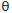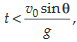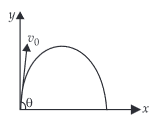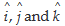# A small particle of mass m is projected at an angle  with the x-axis with an initial velocity v0 in the x-y plane as shown in the figur...

A small particle of mass m is projected at an anglewith the x-axis with an initial velocity v0 in the x-y plane as shown in the figure.

At a timethe angular momentum of the particle isNote:are unit vectors along x, y and z-axis respectively.

Anonymous User Physics Circular and Rotational Motion 12 May, 2020 57 views